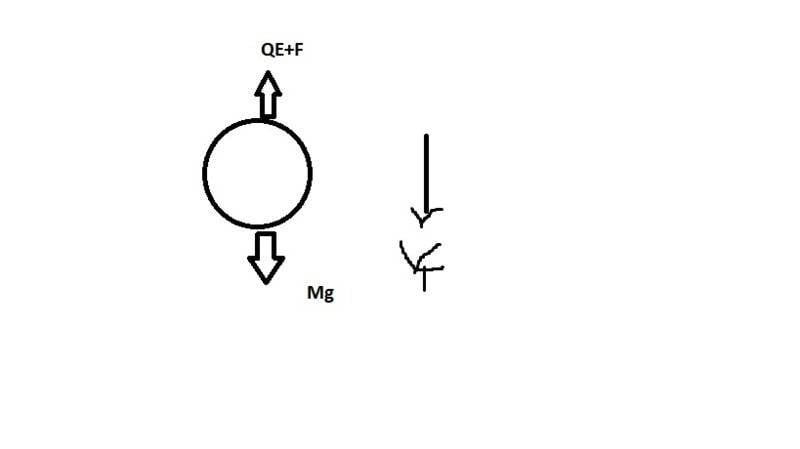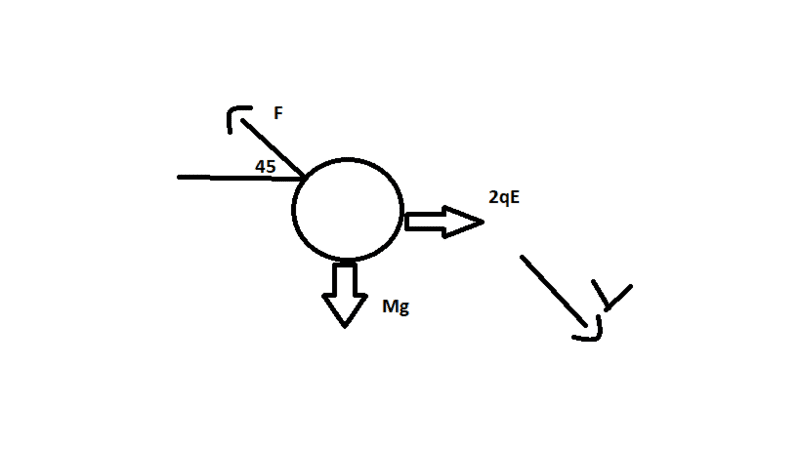# Millikan's oil drop problem

• Krushnaraj Pandya
See my later edit above.Oh, right- I got the correct answer by equating forces in vertical and horizontal directions and plugging into the first. My main mistake was that I considered F to be the same in both cases, Thank you very much for your help :Df

Gold Member

## Homework Statement

In Millikan's oil drop experiment on applying a vertically upward electric field an oil drop (of mass m) moves vertically downward with certain terminal speed. On applying double the electric field in horizontal direction, the drop moves making 45 degrees with the vertical. Neglecting buoyant force due to air, what is the viscous force acting on the drop in first case?

## Homework Equations

Fluid mechanics+electrostatics+mechanics equations

## The Attempt at a Solution

Attached below. From first case, qE+F(viscous force)=mg, from second case F/root2 =mg but this is inconsistent and the answer is mg/2. I'd be grateful for some help#### Attachments

Without the horizontal field it was moving downwards. In your diagram, after adding a horizontal field you have it moving upwards. Does that seem reasonable?

Without the horizontal field it was moving downwards. In your diagram, after adding a horizontal field you have it moving upwards. Does that seem reasonable?
The arrow shows the viscous force, I have it moving downwards. Note the separate arrow pointing southeast shows the velocity.

The arrow shows the viscous force, I have it moving downwards. Note the separate arrow pointing southeast shows the velocity.
Sorry, I should have read it more carefully.
Are you assuming the viscous force is the same in both cases?

In the second diagram, isn't the vertical electric force still acting?
However, to get the answer mg/2 I have to assume it is no longer acting.
What does the fact that it moves at 45 degrees tell you about the applied forces in the second case?

•Krushnaraj Pandya
Sorry, I should have read it more carefully.
Are you assuming the viscous force is the same in both cases?
the viscous force is 6pi*r*n*v. I noticed just now that the velocity might be different in both cases. How can we obtain a relation between them?

the viscous force is 6pi*r*n*v. I noticed just now that the velocity might be different in both cases. How can we obtain a relation between them?
See my later edit above.

Oh, right- I got the correct answer by equating forces in vertical and horizontal directions and plugging into the first. My main mistake was that I considered F to be the same in both cases, Thank you very much for your help :D